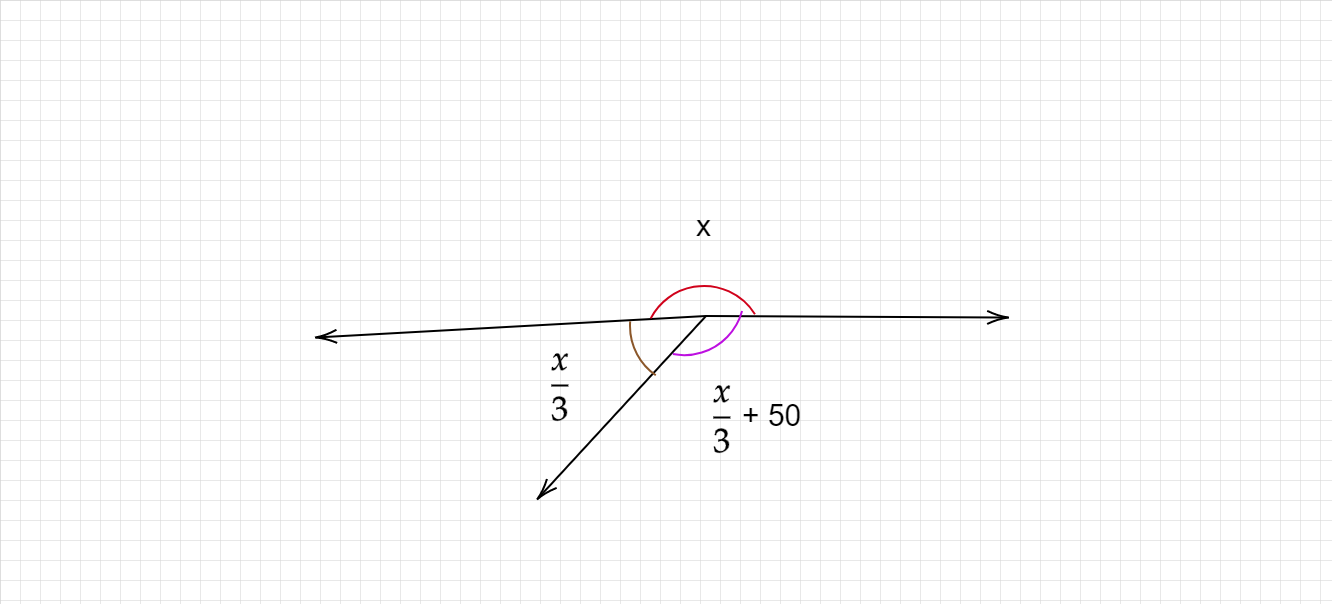"
">

# Find all the angles in the figure given below."

Given :

Angles about a point are $x, \frac{x}{3} and \frac{x}{3} +50$.

To find :

We have to find all the angles in the given figure.

Solution :

We know that,

Sum of the angles about a point is 360°.

$x+ \frac{x}{3} + \frac{x}{3} +50 = 360°$

$\frac{(3x+x+x+3(50°))}{3} = 360°$

$5x+150° = 3(360)°$

$5x+150° = 1080°$

$5x = 1080°-150°$

$5x = 930°$

$x = \frac{930°}{5}$

$x = 186°$

$\frac{x}{3}= \frac{186°}{3} = 62°$

$\frac{x}{3} +50 = \frac{186°}{3} + 50° = 62°+50° = 112°$.

The three angles in the figure are $186° , 62° and 112°$

Updated on: 10-Oct-2022

47 Views